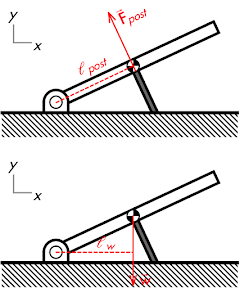## 20141012

### Physics quiz question: diagonally-propped beam

Physics 205A Quiz 5, fall semester 2011
Cuesta College, San Luis Obispo, CA

Cf. Giambattista/Richardson/Richardson, Physics, 2/e, Problems 8.19, 8.35A uniform beam of length 2.7 m is mounted on a pivot at one end, and supported at its center by a post perpendicular to the beam. (Calculate all torques with respect to the pivot.) The magnitude of the force of the post on the beam is __________ the magnitude of the weight of the beam.
(A) less than.
(B) equal to.
(C) greater than.
(D) (Not enough information is given.)

Correct answer (highlight to unhide): (A)The post force on the beam is already acting perpendicular to the beam, so its perpendicular lever armpost is simply L/2.

The weight of the beam acts at its center of gravity, straight downwards. The perpendicular lever arm for the weight force extends from the pivot to perpendicularly intercept the weight force line of action, such that this will be a horizontal line of length:

w = (L/2)·cos25°.

Since the beam is (implied to be) in static equilibrium and does not rotate, then the net torque on it is also equal to zero (Στ = 0), such that the counterclockwise torque of the post on the beam must equal the clockwise torque of weight acting on the beam:

τpost = τw.

Substituting in the perpendicular lever arms for these torques:

Fpost·ℓpost = w·ℓw,

Fpost·(L/2) = w·((L/2)·cos25°),

Fpost = w·cos25°,

Fpost = w·(0.91).

Because the force of the post on the beam has a longer perpendicular lever arm than the perpendicular lever arm of the weight on the beam, then the force of the post on the beam has a smaller magnitude than the weight of the beam.

Sections 70854, 70855
Exam code: quiz05t0rQ
(A) : 15 students
(B) : 25 students
(C) : 10 students
(D) : 0 students

Success level: 29%
Discrimination index (Aubrecht & Aubrecht, 1983): 0.34